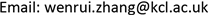1. 研究背景

2. 实证分析

(一) 数据选取

(二) 单位根检验

Unit root test

lngdp−0.437−3.654−2.957−2.617
Δlngdp−4.642−3.670−2.964−2.621
lnser−1.696−3.639−2.951−2.614
Δlnser−3.982−3.646−2.954−2.616
lnind−1.001−3.654−2.957−2.617
Δlnind−3.653−3.662−2.960−2.619
lnagr−1.748−3.627−2.946−2.612
Δlnagr−4.920−3.639−2.951−2.614

(三) Johanson协整检验

(四) Granger因果检验

Granger因果检验是用于分析时间序列变量之间的因果关系的检验方法。假定两组时间序列变量为 [ x t ] 和 [ y t ] ，且有关 [ x t ] 和 [ y t ] 每一变量的预测信息全部包含在这些变量的时间序列当中。构造以下回归方程：

y t = ∑ i = 1 p α i x i − 1 + ∑ j = 1 q β i y i − 1 + μ 1 t

x t = ∑ i = 1 s λ i x i − 1 + ∑ j = 1 r γ i y i − 1 + μ 2 t

Johanson tes

0.49849.73147.8560.031

Cointegration tes

0.9634770.963477
0.9530690.953069
0.763532 − 0.217072i0.793789
0.763532 + 0.217072i0.793789
0.154606 − 0.228228i0.275665
0.154606 + 0.228228i0.275665
0.2263630.226363
0.6640190.664019

(五) 向量自回归(VAR)模型

y t = [ Δ L N ( g d p ) t ,   Δ L N ( arg ) t ,   Δ L N ( i n d ) t ,   Δ L N ( s e r ) t ] ′

y t = A 1 y t − 1 + A 2 y t − 2 + ε t .

Granger causality tes

lnag不是lngdp的GRANGER原因0.7950.565lnagri是lngdp的GRANGER原因
lngdp不是lnag的GRANGER原因3.2930.024
lnind不是lngdp的GRANGER原因0.9480.471lngdp是lnindus的GRANGER原因
lngdp不是lnind的GRANGER原因3.5290.018
lnser不是lngdp的GRANGER原因1.3840.270无GRANGER因果关系
lngdp不是lnser的GRANGER原因1.6850.182
lnind不是lnagr的GRANGER原因1.6140.200Lnagri是lnindus的GRANGER原因
lnagr不是lnind的GRANGER原因2.4520.067
lnser不是lnagr的GRANGER原因4.3970.007Lnser是lnagri的GRANGER原因
lnagr不是lnser的GRANGER原因1.9890.122
lnser不是lnindus的GRANGER原因2.9720.035Lnser是lnindus的GRANGER原因
lnindus不是lnser的GRANGER原因0.7430.600

SC and AIC information criterio

0−12.557−12.59
1−14.081−14.211
2−14.094*−14.271*
3−14.037−14.162
4−13.994−14.023
5−13.788−14.001

(六) 脉冲响应函数

Estimation results of VAR(2) mode
DEL_LNGDPDEL_LNAGRDEL_LNINDDEL_LNSER
DEL_LNGDP(−1)2.0721.5352.4811.097
[1.099][0.579][1.000][0.456]
DEL_LNGDP(−2)4.4612.1405.7345.073
[2.421][0.826][2.363][2.159]
DEL_LNAGR(−1)−0.332−0.205−0.433−0.027
[−0.862][−0.379][−0.855][−0.054]
DEL_LNAGR(−2)0.7850.2941.0111.010
[1.896][0.505][1.855][1.912]
DEL_LNIND(−1)−0.204−0.025−0.1890.042
[−0.232][−0.020][−0.163][0.037]
DEL_LNIND(−2)1.9061.1452.3851.993
[2.164][0.925][2.059][1.775]
DEL_LNSER(−1)0.6690.6680.7970.189
[1.212][0.861][1.097][0.268]
DEL_LNSER(−2)1.1800.4661.6031.316
[2.319][0.651][2.394][2.428]

3. 结论分析

4. 政策建议

(一) 完善法律环境，强化政策支持

(二) 建立统一化的服务标准

(三) 逐步推动服务业市场化进程

(四) 加快行业协会和行业监管机构的建立步伐